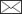Kullervo KuuselaVolume and increment calculation of a sample plot determined with the relascope

Kuusela K. (1960). Volume and increment calculation of a sample plot determined with the relascope. Silva Fennica vol. 71 no. 6 article id 7115. https://doi.org/10.14214/aff.7115

Abstract

The relascope method, introduced by Bitterlich, has been mostly used in estimating the basal area of tree stands or growing stock. Volume estimation requires, in addition, mean height and form. The purpose of this study is to work out a method for calculating the volume, bark and increment of the stand from the measurements of sample trees taken on a plot determined with the relascope. All trees of the same diameter have their own plot size and the stand characteristics are the sum of all tree characteristics multiplied by a blow-up factor which is a function of the diameter.

Accurate determination of a sample plot with the relascope requires checking the boundary trees with a tape. In an average forest there are 10 to 20 unit trees on each plot if the opening of the relascope is 2 cm. Because all trees of equal diameter to be tallied on a sample plot represent an equal share of the total basal area, the number of trees to be tallied is very economical from the stand point of volume estimation. Objective selection of the sample trees can easily be done. The unit volume per hectare represented by each tallied tree, or by each sample tree, is directly proportional to the tree height. Thus, the estimates of the stock characteristics can be calculated as arithmetic means from the sample tree characteristics. The calculation procedure which gives the ordinary stock table, volume, bark and increment is also easily carried out with punched cards.

The PDF includes a summary in Finnish.

Published in 1960

Views 140

Available at https://doi.org/10.14214/aff.7115 |Register
Click this link to register to Silva Fennica.